# RD Sharma Solutions for Class 11 Chapter 28 - Introduction to Three Dimensional Coordinate Geometry Exercise 28.1

Here, in this exercise, we shall discuss concepts related to coordinates of a point in space, signs of coordinates of a point. Students can refer to RD Sharma Class 11 Maths Solutions to secure good marks in the board examination. The solutions are designed by our expert tutors in a step by step manner to help students understand the concepts clearly. Students are advised to practice regularly to come out with flying colours in their board examination. The pdf of RD Sharma Solutions are provided in the links given below, which can be downloaded easily and can be used for future reference as well.

## Download the pdf of RD Sharma Solutions for Class 11 Maths Exercise 28.1 Chapter 28 – Introduction to Three Dimensional Coordinate Geometry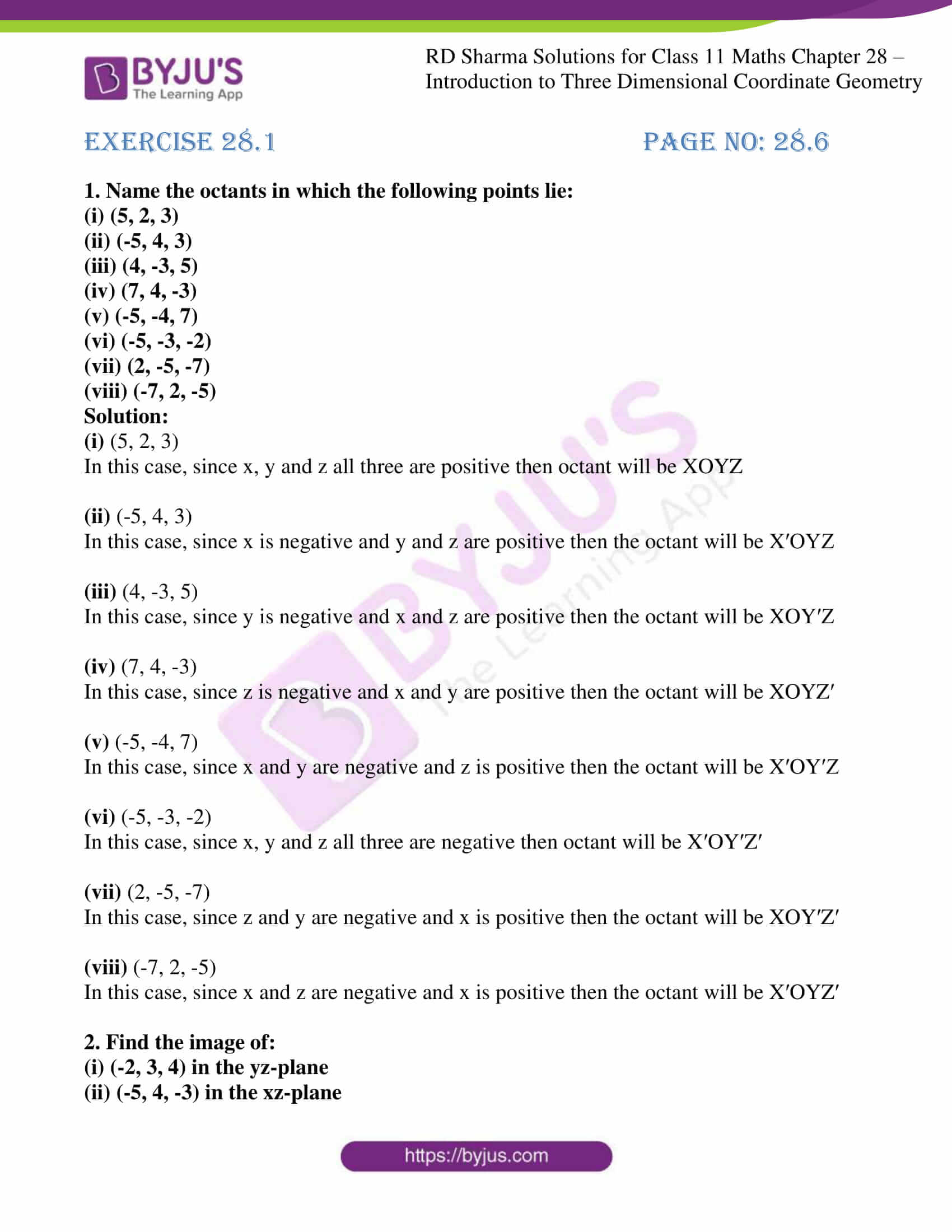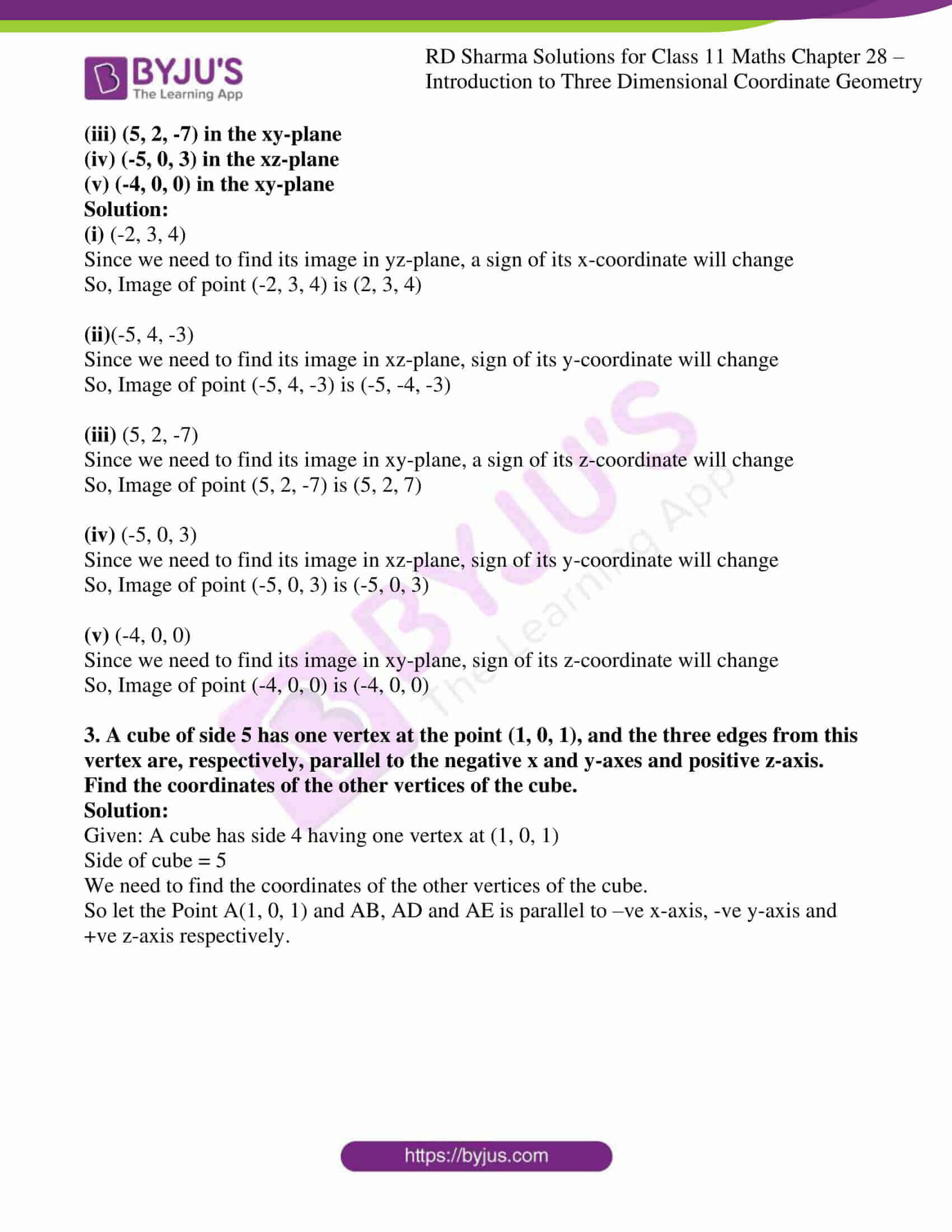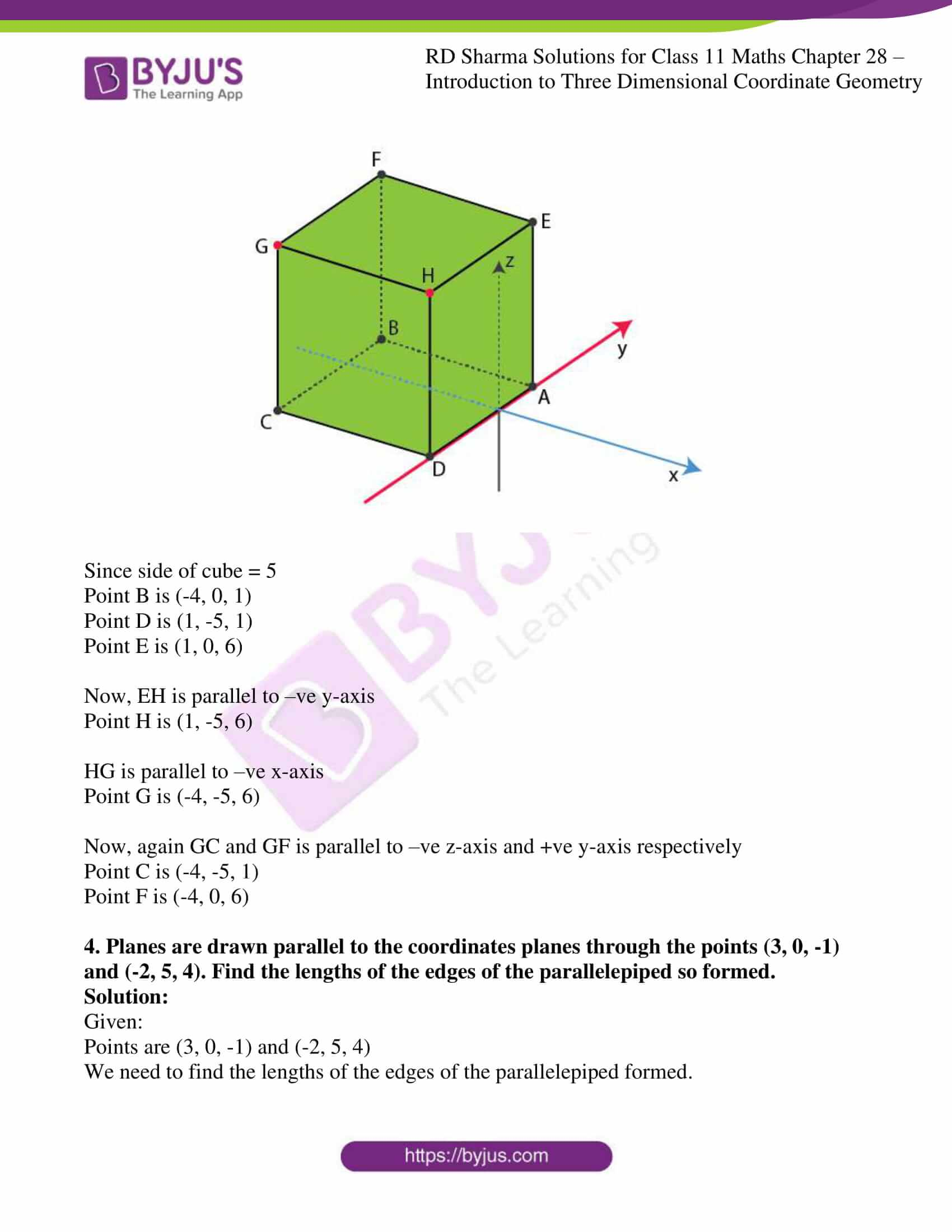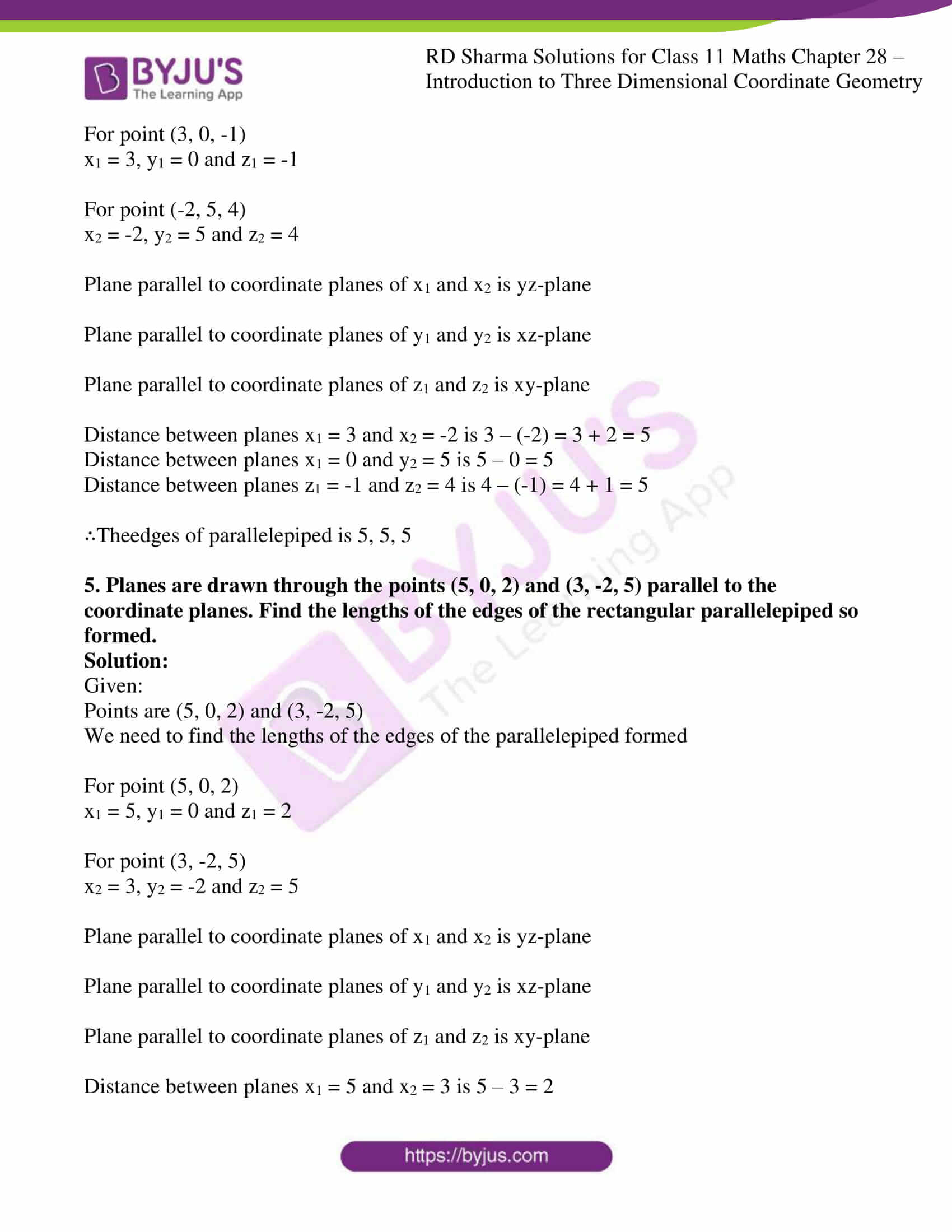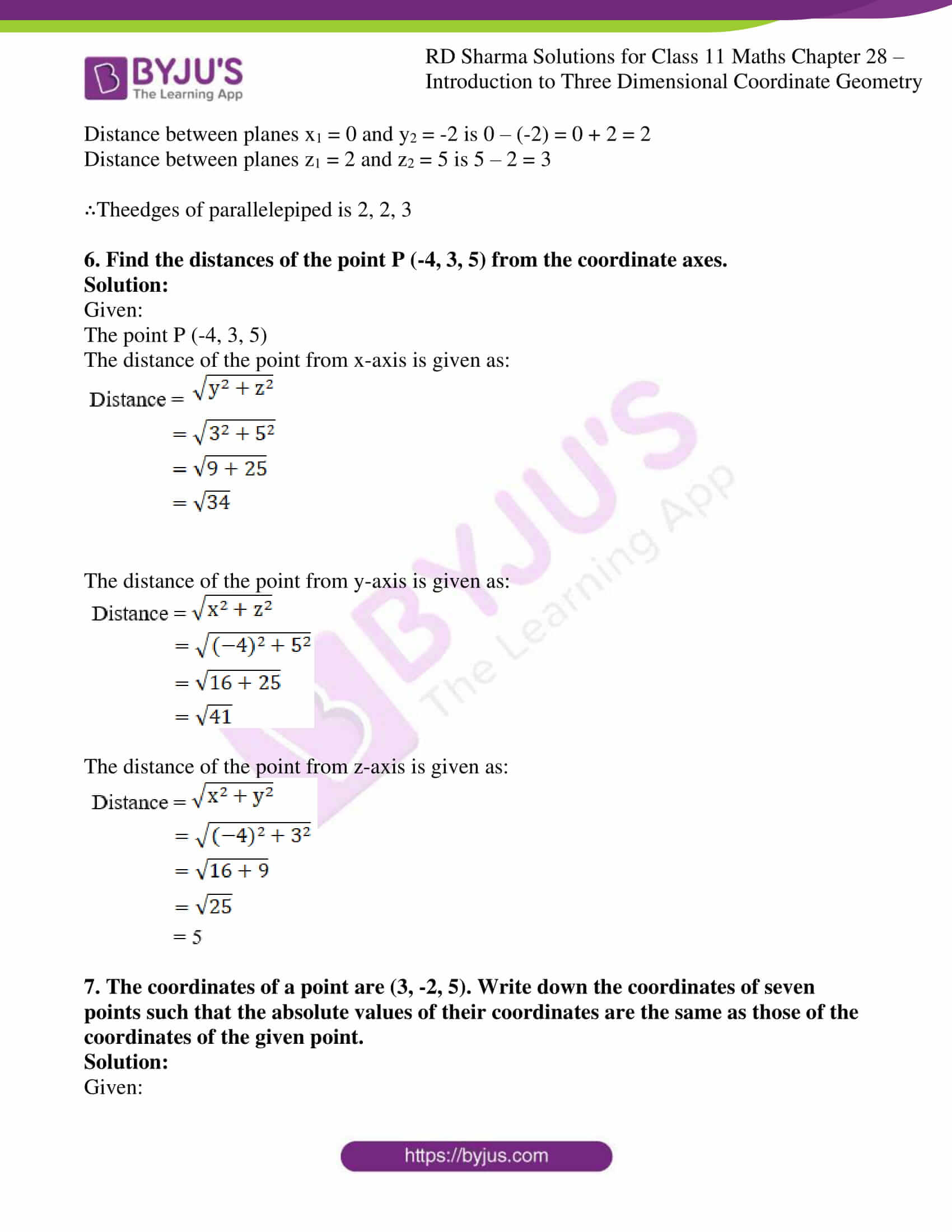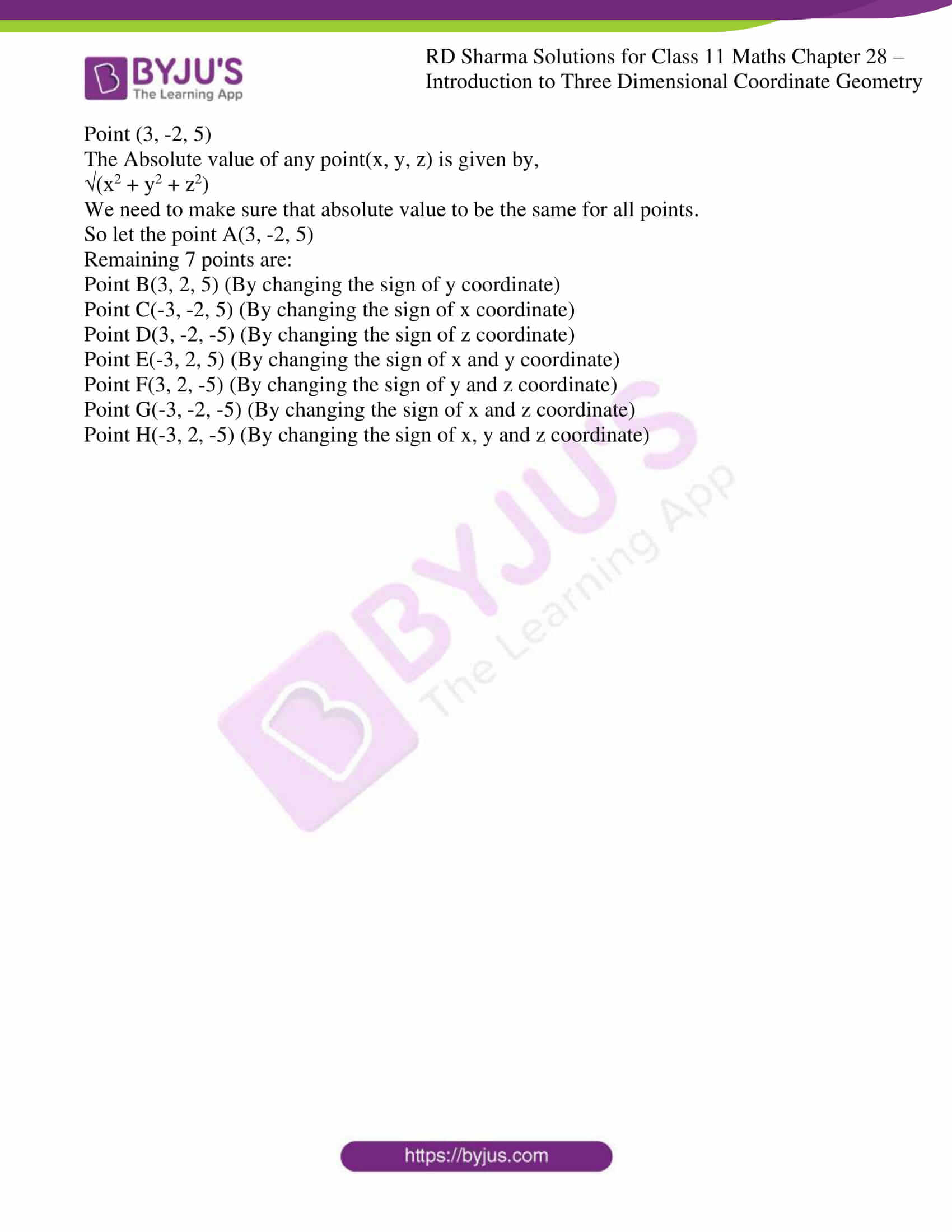### Access answers to RD Sharma Solutions for Class 11 Maths Exercise 28.1 Chapter 28 – Introduction to Three Dimensional Coordinate Geometry

#### ExERCISE 28.1 PAGE NO: 28.6

1. Name the octants in which the following points lie:
(i) (5, 2, 3)
(ii) (-5, 4, 3)
(iii) (4, -3, 5)
(iv) (7, 4, -3)
(v) (-5, -4, 7)
(vi) (-5, -3, -2)
(vii) (2, -5, -7)
(viii) (-7, 2, -5)

Solution:

(i) (5, 2, 3)

In this case, since x, y and z all three are positive then octant will be XOYZ

(ii) (-5, 4, 3)

In this case, since x is negative and y and z are positive then the octant will be X′OYZ

(iii) (4, -3, 5)

In this case, since y is negative and x and z are positive then the octant will be XOY′Z

(iv) (7, 4, -3)

In this case, since z is negative and x and y are positive then the octant will be XOYZ′

(v) (-5, -4, 7)

In this case, since x and y are negative and z is positive then the octant will be X′OY′Z

(vi) (-5, -3, -2)

In this case, since x, y and z all three are negative then octant will be X′OY′Z′

(vii) (2, -5, -7)

In this case, since z and y are negative and x is positive then the octant will be XOY′Z′

(viii) (-7, 2, -5)

In this case, since x and z are negative and x is positive then the octant will be X′OYZ′

2. Find the image of:
(i) (-2, 3, 4) in the yz-plane
(ii) (-5, 4, -3) in the xz-plane

(iii) (5, 2, -7) in the xy-plane
(iv) (-5, 0, 3) in the xz-plane
(v) (-4, 0, 0) in the xy-plane

Solution:

(i) (-2, 3, 4)

Since we need to find its image in yz-plane, a sign of its x-coordinate will change

So, Image of point (-2, 3, 4) is (2, 3, 4)

(ii)(-5, 4, -3)

Since we need to find its image in xz-plane, sign of its y-coordinate will change

So, Image of point (-5, 4, -3) is (-5, -4, -3)

(iii) (5, 2, -7)

Since we need to find its image in xy-plane, a sign of its z-coordinate will change

So, Image of point (5, 2, -7) is (5, 2, 7)

(iv) (-5, 0, 3)

Since we need to find its image in xz-plane, sign of its y-coordinate will change

So, Image of point (-5, 0, 3) is (-5, 0, 3)

(v) (-4, 0, 0)

Since we need to find its image in xy-plane, sign of its z-coordinate will change

So, Image of point (-4, 0, 0) is (-4, 0, 0)

3. A cube of side 5 has one vertex at the point (1, 0, 1), and the three edges from this vertex are, respectively, parallel to the negative x and y-axes and positive z-axis. Find the coordinates of the other vertices of the cube.

Solution:

Given: A cube has side 4 having one vertex at (1, 0, 1)

Side of cube = 5

We need to find the coordinates of the other vertices of the cube.

So let the Point A(1, 0, 1) and AB, AD and AE is parallel to –ve x-axis, -ve y-axis and +ve z-axis respectively.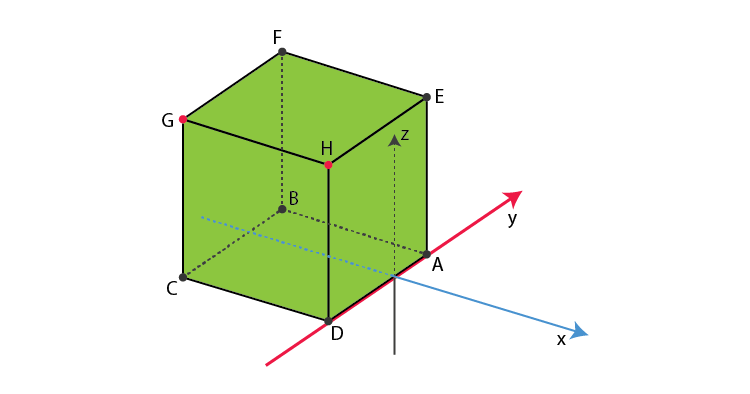Since side of cube = 5

Point B is (-4, 0, 1)

Point D is (1, -5, 1)

Point E is (1, 0, 6)

Now, EH is parallel to –ve y-axis

Point H is (1, -5, 6)

HG is parallel to –ve x-axis

Point G is (-4, -5, 6)

Now, again GC and GF is parallel to –ve z-axis and +ve y-axis respectively

Point C is (-4, -5, 1)

Point F is (-4, 0, 6)

4. Planes are drawn parallel to the coordinates planes through the points (3, 0, -1) and (-2, 5, 4). Find the lengths of the edges of the parallelepiped so formed.

Solution:

Given:

Points are (3, 0, -1) and (-2, 5, 4)

We need to find the lengths of the edges of the parallelepiped formed.

For point (3, 0, -1)

x1 = 3, y1 = 0 and z1 = -1

For point (-2, 5, 4)

x2 = -2, y2 = 5 and z2 = 4

Plane parallel to coordinate planes of x1 and x2 is yz-plane

Plane parallel to coordinate planes of y1 and y2 is xz-plane

Plane parallel to coordinate planes of z1 and z2 is xy-plane

Distance between planes x1 = 3 and x2 = -2 is 3 – (-2) = 3 + 2 = 5

Distance between planes x1 = 0 and y2 = 5 is 5 – 0 = 5

Distance between planes z1 = -1 and z2 = 4 is 4 – (-1) = 4 + 1 = 5

∴Theedges of parallelepiped is 5, 5, 5

5. Planes are drawn through the points (5, 0, 2) and (3, -2, 5) parallel to the coordinate planes. Find the lengths of the edges of the rectangular parallelepiped so formed.

Solution:

Given:

Points are (5, 0, 2) and (3, -2, 5)

We need to find the lengths of the edges of the parallelepiped formed

For point (5, 0, 2)

x1 = 5, y1 = 0 and z1 = 2

For point (3, -2, 5)

x2 = 3, y2 = -2 and z2 = 5

Plane parallel to coordinate planes of x1 and x2 is yz-plane

Plane parallel to coordinate planes of y1 and y2 is xz-plane

Plane parallel to coordinate planes of z1 and z2 is xy-plane

Distance between planes x1 = 5 and x2 = 3 is 5 – 3 = 2

Distance between planes x1 = 0 and y2 = -2 is 0 – (-2) = 0 + 2 = 2

Distance between planes z1 = 2 and z2 = 5 is 5 – 2 = 3

∴Theedges of parallelepiped is 2, 2, 3

6. Find the distances of the point P (-4, 3, 5) from the coordinate axes.

Solution:

Given:

The point P (-4, 3, 5)

The distance of the point from x-axis is given as: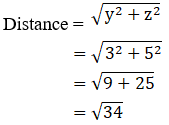The distance of the point from y-axis is given as: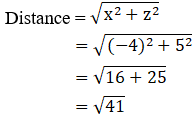The distance of the point from z-axis is given as: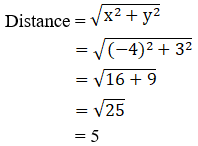7. The coordinates of a point are (3, -2, 5). Write down the coordinates of seven points such that the absolute values of their coordinates are the same as those of the coordinates of the given point.

Solution:

Given:

Point (3, -2, 5)

The Absolute value of any point(x, y, z) is given by,

√(x2 + y2 + z2)

We need to make sure that absolute value to be the same for all points.

So let the point A(3, -2, 5)

Remaining 7 points are:

Point B(3, 2, 5) (By changing the sign of y coordinate)

Point C(-3, -2, 5) (By changing the sign of x coordinate)

Point D(3, -2, -5) (By changing the sign of z coordinate)

Point E(-3, 2, 5) (By changing the sign of x and y coordinate)

Point F(3, 2, -5) (By changing the sign of y and z coordinate)

Point G(-3, -2, -5) (By changing the sign of x and z coordinate)

Point H(-3, 2, -5) (By changing the sign of x, y and z coordinate)